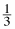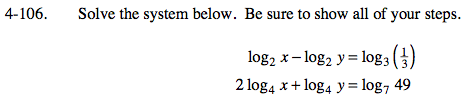### Home > PC > Chapter 4 > Lesson 4.2.3 > Problem4-106

4-106.

Solve the system below. Be sure to show all of your steps. Homework Help ✎

log2 x − log2 y = log3 ()
2 log4 x + log4 y = log7 49Simplify the first equation.

$\text{log}_2\frac{x}{y}=\text{ log}_33^{-1}$

$\text{log}_2\frac{x}{y}=-1$

$2^-^1=\frac{x}{y}$

$\frac{1}{2}=\frac{x}{y}$

$y=2x$

Simplify the second equation.

$\text{log}_{4}x^{2}y=\text{ log}_{7}7^2$

$\text{log}_{4}x^{2}y=2$

$4^2=\textit{x}^2\textit{y}$

$16=\textit{x}^2\textit{y}$

Substitute:

$16=2\textit{x}^3$

$8=x^3$

$x=2$

$y = 2x$

$y=2(2)$

$y=4$